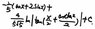# Integrating $\int {\frac{sin^{2}x}{1+sin^{2}x}dx}$ Solution

• foxtrot1985

## Homework Statement

$$\int {\frac{sin^{2}x}{1+sin^{2}x}dx}$$

## Homework Equations

Let t = tan x/2, then dx = 2/(1+t^2) and sin x = 2t / (1+t^2)

## The Attempt at a Solution

I got up to the point where $$\int {\frac{8t^{2}}{(1+6t^{2}+t^{4})(1+t^{2})} dt}$$. Not sure if I'm on the right track and if I am, do I use partial fractions after this?

The final answer is attached. Can't really make out the handwriting :/

#### Attachments

•8.9 KB · Views: 329
Last edited:
Yes, it looks like partial fractions is the way to go after your substitution.

Hmm after I do partial fractions, I get
$$\int {\frac{2}{1+t^{2}} + {\frac{-2t^{2}-2}{(1+6t^{2}+t^{4})} dt}$$

After this, I do not know what's the next step. Kindly advise. Thanks.

you are summing 2 functions of t , one of these two look very much like a derivative of a certain function..

If you mean 2tan^-1 t, I can get this part. But what about the 2nd function?